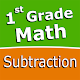Home » Educational » First grade Math - Subtraction# First grade Math - Subtraction

Sergey Malugin

(11)
Math facts fluency practice app with handwriting input and cool & fun mini game.

## The description of First grade Math - Subtraction

Intuitive interface powered by handwriting input and a fun and engaging mini game in addition to a regular math trainer mode make our app stand out from the crowd of generic math learning apps.

With First grade Math - Subtraction you can practice and improve the following math skills:
- Subtraction facts - numbers up to 10
- Subtraction facts - numbers up to 18
- Subtraction facts - numbers up to 20
- Subtract multiples of 10
- Subtract a multiple of ten
- Subtract one-digit number from two-digit number
- Subtract two two-digit numbers

Choose between two different modes:
- Math Trainer
- Math Shot mini-game

Math Trainer mode helps you to master the math facts, you can solve math problems at you own pace without any time constrain.

Math Shot is a math mini-game with fun and engaging game-play and as we all know learning through play and fun is more effective. The game difficulty adapts to player's skills and makes the game suitable for all ages. Math Shot helps students to develop math fact fluency - the result of consistent learning, repetition, and practice.
Publish Date: April 02, 2018